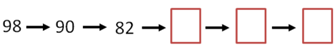Mathematics
Easy

Question

# What are the next three numbers in given sequences by following the rule?Rule: subtract 8## 76, 68, 60    74, 68, 60    74, 66, 58    72, 64, 56Hint:

## The correct answer is: 74, 66, 58

### The given sequence is 98, 90, 82We have to find the next three terms in this sequence.Rule: Subtract 8To find the next term, 8 is subtracted from the previous term.We will see how it works.98 – 8 = 9090 – 8 = 82Now, let’s find the next terms.The last term from the above sequence is 82So, we will subtract 8 from it.82 – 8 = 7474 – 8 = 6666 – 8 = 58The complete sequence will be 98, 90, 82, 74, 66, 58And the option which is '74, 66, 58’, is the required option.

For such questions, we should know the basic operations. When the rule used to write the pattern is not mentioned, we have to use all four operations to check the pattern. The operations are addition, subtraction, division, and multiplication.

### Related Questions to study#### With Turito Foundation.#### Get an Expert Advice From Turito.